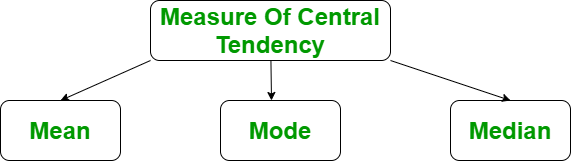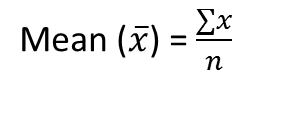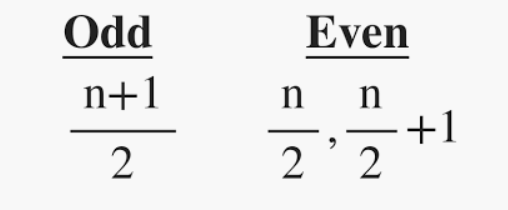Open in App
Not now

## Related Articles

• Write an Interview Experience

# Mean, Median and Mode in R Programming

• Difficulty Level : Easy
• Last Updated : 15 Nov, 2022

The measure of central tendency in R Language represents the whole set of data by a single value. It gives us the location of central points. There are three main measures of central tendency

• Mean
• Median
• ModePrerequisite

Before doing any computation, first of all, we need to prepare our data, save our data in external .txt or .csv files and it’s a best practice to save the file in the current directory. After that import, your data into R as follow:

Get the CSV file here.

## R

 `# R program to import data into R` `# Import the data using read.csv()``myData = ``read.csv``(``"CardioGoodFitness.csv"``,``                  ``stringsAsFactors=F)``# Print the first 6 rows``print``(``head``(myData))`

Output:

```   Product Age Gender Education MaritalStatus Usage Fitness Income Miles
1   TM195  18   Male        14        Single     3       4  29562   112
2   TM195  19   Male        15        Single     2       3  31836    75
3   TM195  19 Female        14     Partnered     4       3  30699    66
4   TM195  19   Male        12        Single     3       3  32973    85
5   TM195  20   Male        13     Partnered     4       2  35247    47
6   TM195  20 Female        14     Partnered     3       3  32973    66```

## Mean in R Programming Language

It is the sum of observations divided by the total number of observations. It is also defined as average which is the sum divided by count.Where, n = number of terms

Example:

## R

 `# R program to illustrate``# Descriptive Analysis` `# Import the data using read.csv()``myData = ``read.csv``(``"CardioGoodFitness.csv"``,``                  ``stringsAsFactors=F)` `# Compute the mean value``mean = ``mean``(myData\$Age)``print``(mean)`

Output:

` 28.78889`

## Median in R Programming Language

It is the middle value of the data set. It splits the data into two halves. If the number of elements in the data set is odd then the center element is median and if it is even then the median would be the average of two central elements.Where n = number of terms

Syntax: median(x, na.rm = False)

Where, X is a vector and na.rm is used to remove missing value

Example:

## R

 `# R program to illustrate``# Descriptive Analysis` `# Import the data using read.csv()``myData = ``read.csv``(``"CardioGoodFitness.csv"``,``                  ``stringsAsFactors=F)` `# Compute the median value``median = ``median``(myData\$Age)``print``(median)`

Output:

` 26`

## Mode in R Programming Language

It is the value that has the highest frequency in the given data set. The data set may have no mode if the frequency of all data points is the same. Also, we can have more than one mode if we encounter two or more data points having the same frequency. There is no inbuilt function for finding mode in R, so we can create our own function for finding the mode or we can use the package called modeest.

### Creating user-defined function for finding Mode

There is no in-built function for finding mode in R. So let’s create a user-defined function that will return the mode of the data passed. We will be using the table() method for this as it creates a categorical representation of data with the variable names and the frequency in the form of a table. We will sort the column Age column in descending order and will return the 1 value from the sorted values.

Example: Finding mode by sorting the column of the dataframe

## R

 `# Import the data using read.csv()``myData = ``read.csv``(``"CardioGoodFitness.csv"``,``                  ``stringsAsFactors=F)` `mode = ``function``(){``    ``return``(``sort``(-``table``(myData\$Age)))``}` `mode``()`

Output:

`25: -25`

### Using Modeest Package

We can use the modeest package of the R. This package provides methods to find the mode of the univariate data and the mode of the usual probability distribution.

Example:

## R

 `# R program to illustrate``# Descriptive Analysis` `# Import the library``library``(modeest)` `# Import the data using read.csv()``myData = ``read.csv``(``"CardioGoodFitness.csv"``,``                  ``stringsAsFactors=F)` `# Compute the mode value``mode = ``mfv``(myData\$Age)``print``(mode)`

Output:

` 25`

My Personal Notes arrow_drop_up